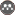Volume 11, Issue 41 (fall 2007)                   JWSS 2007, 11(41): 29-42 | Back to browse issues page

BibTeX | RIS | EndNote | Medlars | ProCite | Reference Manager | RefWorks
Send citation to:A. Majnooni-Heris, Sh. Zand - Parsa, A. R. Sepaskhah, A. A. Kamgar-Haghighi. Comparison of MSM Model for Prediction of Potential Evapotranspiration of Maize with FAO Methods. JWSS. 2007; 11 (41) :29-42
URL: http://jstnar.iut.ac.ir/article-1-724-en.html
Abstract:   (21260 Views)
Optimal crop water requirement is needed for precise irrigation scheduling. Prediction of crop water requirements is a basic factor to achieve this goal. In this study, maize potential evapotranspiration (ETp) was prediced by maize simulation model (MSM). Then, it was evaluated and validated using experimental field data obtained in Agricultural Research Station of Shiraz University (Bajghah, Fars province) during 2003 and 2004. Comparison of measured volumetric soil water content with predicted values by MSM model in 2003 and 2004 indicated that this subroutine (prediction of maize evapotranspiration) did not need modification. Also, daily potential evapotranspiration of maize was estimated by using Penman-Monteith equation considering single and dual crop coefficients. Comparison between the results of predicted ETp by MSM model, calculated ETp by Penman-Monteith, and measured irrigation water and soil water content indicated that the prediction of ETp by MSM model was satisfactory. Model prediction of seasonal ETp, potential transpiration (Tp) and soil evaporation (E) were 831, 536 and 329 mm, respectively, in 2003, and 832, 518 and 314 mm, respectively, in 2004. The values of ETp, Tp and E calculated by Penman-Monteith method using dual crop coefficients were 693, 489 and 205 mm, respectively, in 2003, and 700, 487 and 213, respectively in 2004. Maximum rate of predicted potential ETp, Tp and E were 11.1, 8.2 and 5.1 mm d-1, respectively in 2003 and 13.0, 9.0 and 4.0 mm d-1, respectively in 2004. The values of calculated seasonal ETp by Penman-Monteith method using single crop coefficient were 615 and 632 mm in 2003, and 2004, respectively. Comparison between the results of predicted ETp by MSM model, calculated ETp by Penman-Monteith equation with single and dual crop coefficients (FAO-56) and measured values of irrigation water and soil water contents of root depth indicated that FAO-56 methods underestimated the ETp.